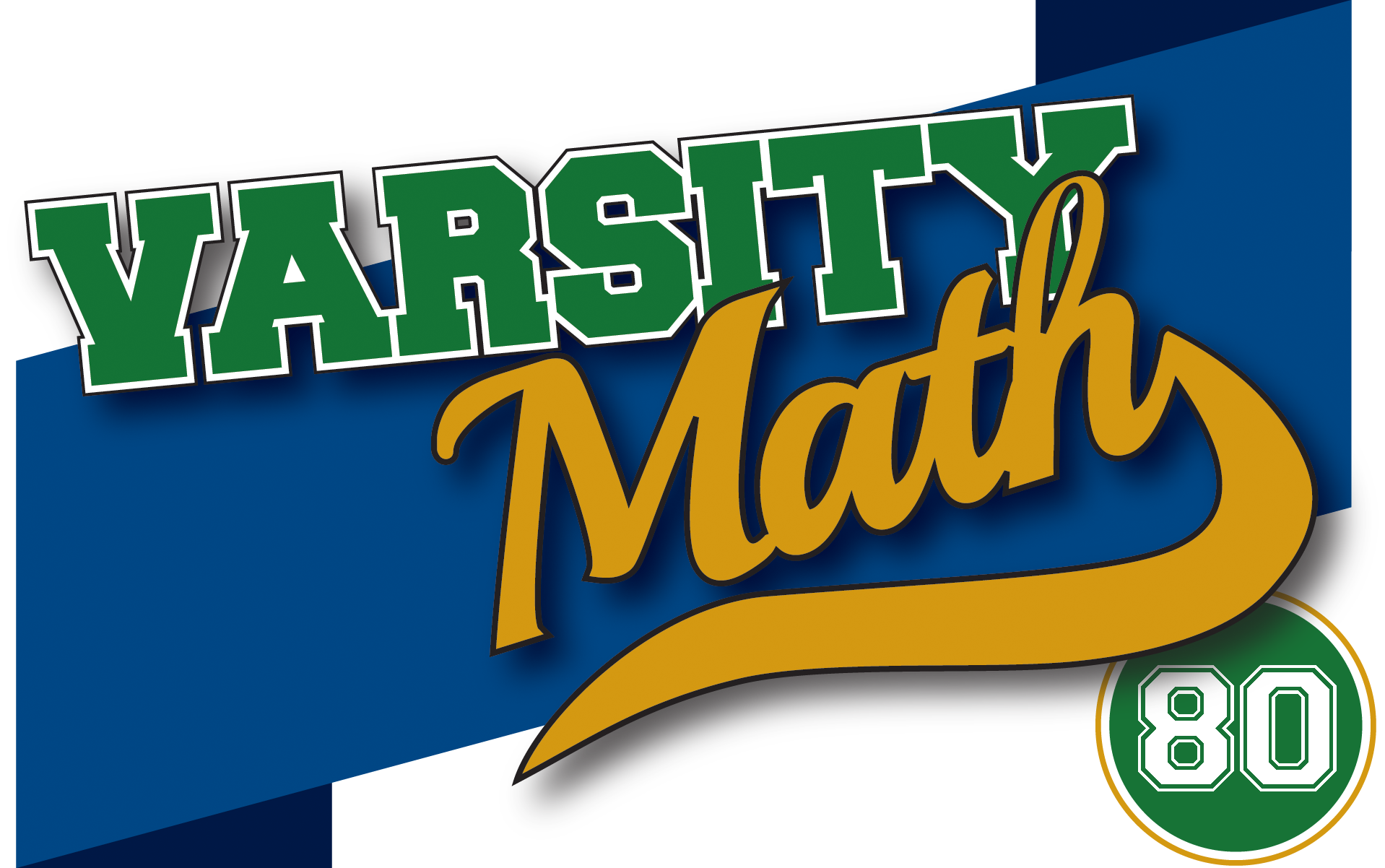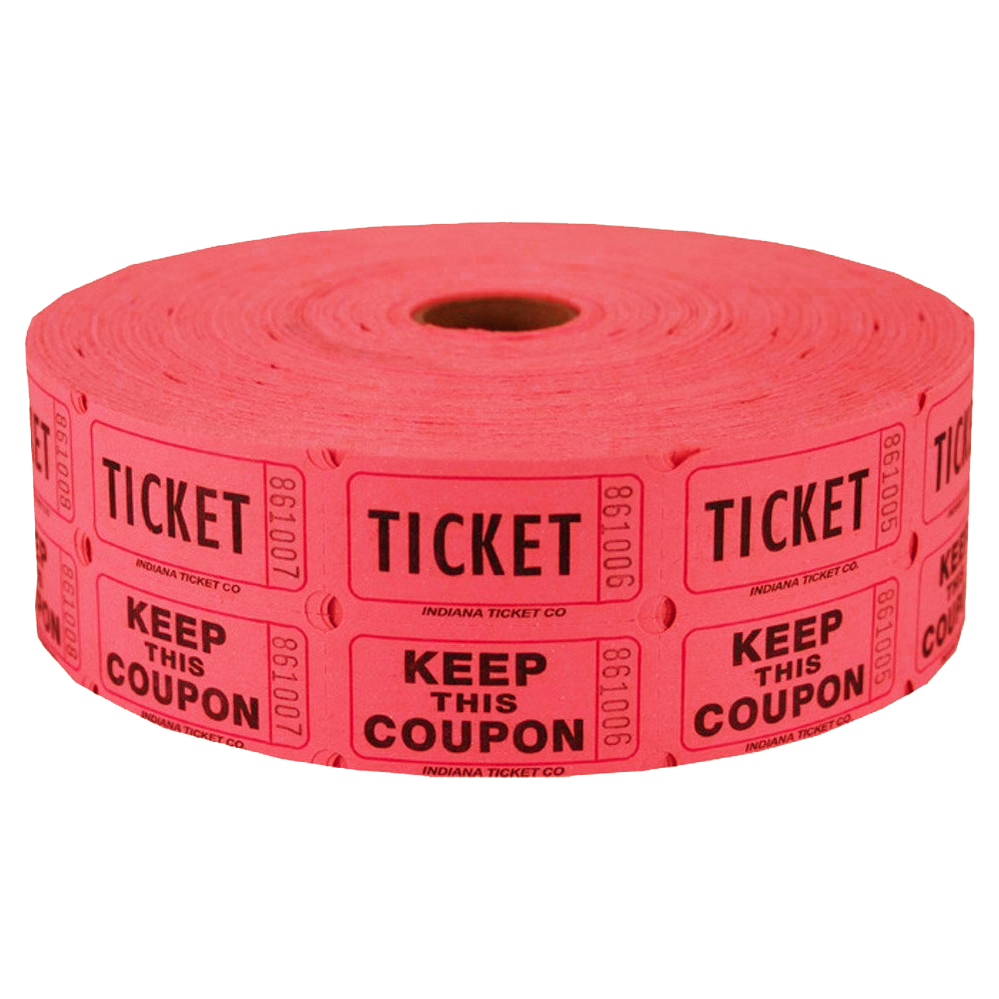## ________________

In light of his recent birthday, Coach Newton decides that it is time to retire at the end of this academic year. “Oh no!” cry the students. Coach Newton responds, “Don’t worry, Coach Taylor will be helping out for the rest of the semester, and she will continue coaching with the excellence shown by this first pair of her puzzles.”

## ________________

### Secret Remainder

Coach Taylor says, “I start with the perfect square of an integer, subtract one, and divide by eight (with no remainder) to produce my secret number, a whole number S. S is not divisible by three.”

What is the remainder when S is divided by nine?### Party Puzzle

Coach Taylor tells about a party she went to where the hosts gave a door prize in an unusual way. All N guests arranged themselves in a circle and counted off clockwise, with person two just to the left of person one, and person three to the left of person two, and so on all the way around so that person one is just to the left of person N. The host then started in front of person one and handed out red tickets as follows: The host skipped one person who did not have a red ticket (and any people who already had a red ticket) and gave a red ticket to the second person around the circle clockwise who did not have one, then repeated this process from the new position around the circle. So for example, the first red ticket went to person two, and the next one went to person four. This process continued until only one person did not have a red ticket, who then got the door prize.

If N is the smallest number of partygoers so that person eleven gets the door prize, what is N?

## Solutions to week 79

Wing Nuts. Let o be the number of ostriches purchased, e be the number of emus, and p be the number of puffins. It’s easy to get two equations: o + e + p = 100, and 10o + 5e + p/2 = 100. That doesn’t seem like enough equations, though, since there are three unknowns. Nevertheless, we may as well try eliminating p by doubling the second equation and subtracting the first, to get 19o + 9e = 100. Since 19×6 > 100, the only possibilities for o are 0, 1, 2, 3, 4, and 5. Trying each in turn, the only value of o that leads to a solution for e is o = 1, which gives e = 9. Since that accounts for ten birds, we know that you must buy 90 puffins to round out the flock. Thus, the only way to buy 100 birds for \$100 is 1 ostrich, 9 emus, and 90 puffins.

Seven Eleven. Again, if we let a, b, c, and d be the four prices, it is easy to get two equations, which are a+b+c+d = 7.11, and abcd = 7.11, respectively. But now there are four unknowns, so two equations seems like hopelessly too few. But anyhow, to forge ahead, it seems like it might be helpful to get the fractions out by switching to the prices in cents, whole numbers A, B, C, and D corresponding to a, b, c, and d, respectively. (Specifically, A = 100a and so on.)

Then our two equations become A+B+C+D = 711 and ABCD = 711,000,000. Since all of the prices are positive, we know that each price is 708 cents or less. This in turn means that each price is 3 cents or more (since 711,000,000 / 708³ > 2), so in fact each price is 702 cents or less. Furthermore, each price in cents is a factor of 711,000,000, since that is their product. In addition, 711,000,000 has a factor of 79, which is prime, so one of the four prices (say D) must be a multiple of 79 cents, between 3 and 702, or in other words, 79, 158, 237, 316, 395, 474, or 632 cents. Suppose it was 632. Then we would have A+B+C = 79, but ABC = 1,125,000, which is impossible since even 79 cubed is only 493,039.

To eliminate more options, recall that the largest area rectangle with a given perimeter is a square; in other words, the largest the product of two numbers with given sum can be occurs when the two numbers are equal. The same principle holds for three numbers. So if one of the prices were 474 cents, then we would have A+B+C = 237, but ABC = 1,500,000, which is impossible because the largest possible product of three numbers summing to 237 is 79 cubed, which again is only 493,039. And in fact, this technique just manages to eliminate 395 cents as well, because then we would have A+B+C = 316 with ABC = 1,800,000, which is impossible because (316/3)³ is only 1,168,685 and a fraction (1/27 to be exact).

Thus, the largest possible price for the item which is a multiple of 79 cents is 316 cents. Let’s see if we can get that to work. We then have A+B+C = 395, while ABC = 2,250,000. Since the cube of 395/3 is just a little bit larger than 2,250,000, it stands to reason that if there is such a solution, all of A, B, and C would have to be pretty near 131 2/3 (=395/3). Listing the factors of 2,250,000, the four nearest to 131 are 120, 125, 144, and 150. We notice that the three of these that are multiples of five, namely 120, 125, and 150, sum to 395, and serendipitously, 120×125×150 = 2,250,000. So we conclude that the prices of the four items are \$1.20, \$1.25, \$1.50, and \$3.16. (You can double check that those four numbers both sum and multiply to 7.11.)

Coach Newton warns that you need to do more work to show this is is the only solution, namely demonstrate why you can’t have an item with price \$2.37, \$1.58, or \$0.79. But it does turn out that none of those prices can work; this really is the only solution.

## Recent Weeks

Links to all of the puzzles and solutions are on the Complete Varsity Math page.

Come back next week for answers and more puzzles.

[asciimathsf]